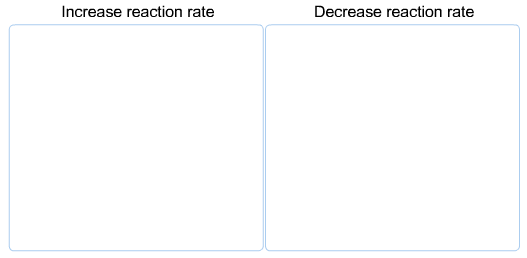# Problem: How would the following events affect the rate of the following general reaction? A + BC → ABCincreasing the temperatureincreasing the concentration of BCadding a catalystdecreasing the concentration of A

###### FREE Expert Solution

Identify how the following events affect the rate of the following general reaction:

A + BC  ABC

A. increasing the temperature

• temperature increases the kinetic energies of molecules, thus increases the rate
• Increase reaction rate

80% (495 ratings)###### Problem Details

How would the following events affect the rate of the following general reaction?

A + BC → ABCincreasing the temperature

increasing the concentration of BC